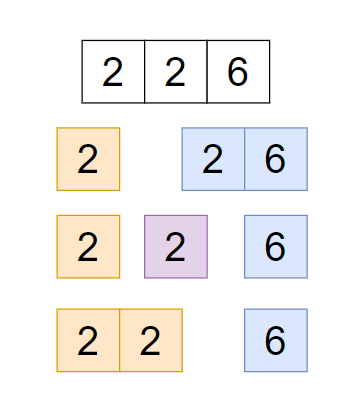# Decode Ways

Posted: 13 Jan, 2021
Difficulty: Moderate

## PROBLEM STATEMENT

#### Example :#### Input format :

``````The first line of input contains an integer ‘T’ denoting the number of test cases. The 'T' test cases follow.

The first line of each test case contains a single string ‘strNum’.
``````

#### Output Format :

``````For each test case, print an integer denoting the ways of decodes.
``````

#### Note :

``````1. You do not need to print anything, it has already been taken care of. Just implement the given function.
2. Ways of decodes can be very high, so return answer modulo 10^9+7.
``````

#### Constraints :

``````1 <= T <= 50
1 <= N <= 10^4

Time limit: 1 second
``````Approach 1

The basic idea is that, try to use each and every possible combination.

• Use a function ‘decodeWaysHelper(strNum,n)’, where ‘strNum’ is a given number as a string, ‘n’ is the size of ‘strNum’. And ‘decodeWaysHelper’ returns the number of decode ways.
• If ‘n==0’ or ‘n==1’, then return ‘1’, because a single number can form only one character/ one way.
• If ‘strNum[n-1]’ is ‘0’ then return ‘0’ because we don't have a mapping of ‘0’ with any character.
• Else use a variable ‘count=0’ to store answer for first ‘n’ number of ‘strNum’
• called ‘decodeWaysHelper(strNum,n-1)’, stored the result in the ‘count’ variable, because the last digit can be from ‘1’ to ‘9’.
• If ‘strNum[n-2]==1’ then call ‘decodeWaysHelper(strNum,n-2)’ because last two digits can be from ‘11’ to ‘19’
• If ‘strNum[n-2]==2 and strNum[n-1]<7’ then call ‘decodeWaysHelper(strNum,n-2)’ because the last two digits can be from ‘20’ to ‘26’.
• At the end return count.